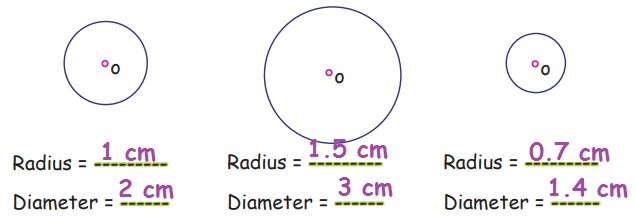Home | | Maths 4th Std | Identifying center, radius and diameter of a circle

# Identifying center, radius and diameter of a circle

Circle is perfectly round in shape. It has no sides and no diagonals. 'O' is the center of the circle. The distance from the center to each of these points A, B, C, D, E is the radius of the circle.

Identifying center, radius and diameter of a circle

Circle is perfectly round in shape. It has no sides and no diagonals. 'O' is the center of the circle. The distance from the center to each of these points A, B, C, D, E is the radius of the circle.

Radius is equal in length (OA=OB=OC=OD=OE).

All the radii are equal in length in a circle.A Line segment AB passes through the center of the circle O. AB is the diameter of the circle. The line segments XY and LM are the Chords (XY, LM, AB).

The longest chord of a circle is the diameter (AB). The radius is always half of the diameter.

Diameter is the longest chord.EXAMPLE

1. Find the diameter of a circle whose radius is 5 cm.

= 2 x 5

Diameter = 10 cm

2. Find the radius of a circle whose diameter is 88cm.

Radius = diameter / 2 = 88 / 2

Activity

Measure the radius and diameter of the following circles.Tags : Geometry | Term 1 Chapter 1 | 4th Maths , 4th Maths : Term 1 Unit 1 : Geometry
Study Material, Lecturing Notes, Assignment, Reference, Wiki description explanation, brief detail
4th Maths : Term 1 Unit 1 : Geometry : Identifying center, radius and diameter of a circle | Geometry | Term 1 Chapter 1 | 4th Maths